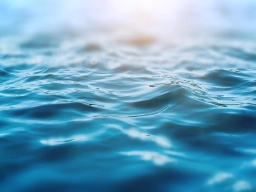# Energy 5

Energy is measured using the unit Joule (J). Temperature is measured using the unit degree Celsius °C). To increase the temperature of 1 liter of water by 1°C, 4200 J of heat energy is required.

(a) Kelly wants to increase the temperature of 2 liters of water from 25°C to 100°C.

(1) Find the amount of heat energy required.
(ii) The energy efficiency of an electric kettle A is 60%; that is, it requires 1 J of electrical energy to produce 0.6 J of heat energy. Find the amount of electrical energy kettle A requires.

(b) Another electric kettle B requires 80% of the electrical energy used by electric kettle A. Find the energy efficiency of electric kettle B.

Q1 =  630000 J
Q2 =  1050000 J
η2 =  75 %

### Step-by-step explanation:Did you find an error or inaccuracy? Feel free to write us. Thank you!

Tips for related online calculators